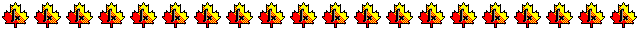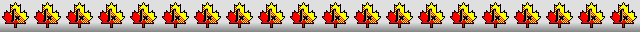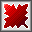Maple worksheets on floating-point arithmetic and errorsNumerical methods topics:

They are all compatible with Classic Worksheet Maple 10.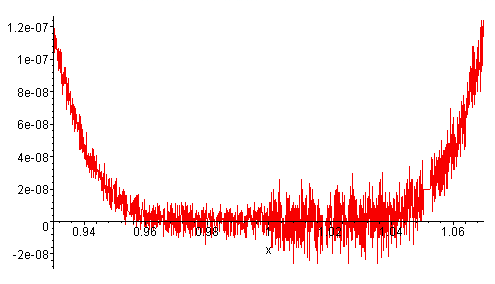Maple's floating-point arithmetic - float.mws

• Floating-point numbers and the precision of computer arithmetic.
• Rounding and significant figures.
• Errors caused by rounding.
• Exact versus floating point arithmetic.
• Maple's hardware and software floating point arithmetic.
• The machine epsilon.
• A unit in the last place .. 1 ulp

Errors in computer floating point arithmetic - errors.mws

• Absolute and relative error.
• Rounding errors.
• Absolute and relative errors in relation to ulp's and the machine epsilon.
• The accumulation of rounding errors.
• Reducing rounding errors in a sum of positive terms.

Graphical illustration of the accumulation of rounding errors - errors2.mws

• An example to illustrate the accumulation of rounding errors graphically.
• Another example to illustrate the accumulation of rounding errors.

Subtraction round-off errors - errors3.mws

• Subtraction round-off error.
• Avoiding subtraction round-off errors.
• A family of integrals whose evaluation involves subtraction errors.
• Upward and downward  recursion.
• Subtraction round-off error in the evaluation of ln(1-x)/x when x is near 0.
• Subtraction round-off error in the evaluation of (1-cos x)/x^2 when x is near 0.

Taylor series - taylor.mws

• Finding Taylor series and Taylor polynomials with Maple.
• Using Taylor polynomials to approximate functions.

Taylor series approximations for the exponential function - expsrs.mws

• Graphing partial sums of a Taylor series.
• Truncation error in computing partial sums.
• Writing a Maple procedure to evaluate the exponential function using partial sums of a Taylor series.

EVALUATION OF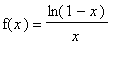USING HARDWARE FLOATING POINT ARITHMETIC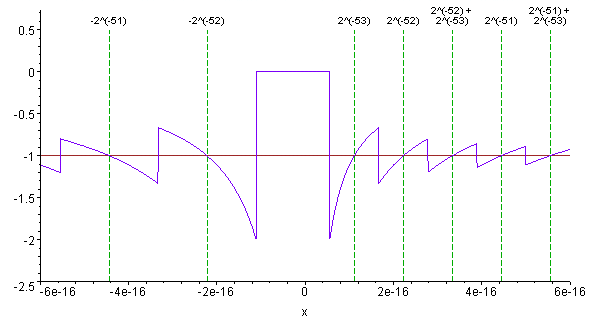Top of page

Main index